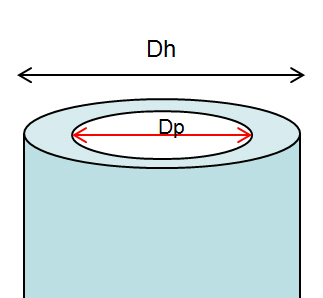# Calculate Annular Capacity

Annular capacity  is volume of fluid between two diameter of cylindrical objects per length or length per volume. This article demonstrates you how to calculate annular capacity between casing or hole and drill pipe, tubing, or casing. There are several formulas as shown below to calculate annular capacity depending on unit of annular capacity required.Note: Dh is bigger ID and Dp is smaller OD. The examples below will show the Dh as hole size and Dp is drill pipe OD## a) Calculate annular capacity in bbl/ft

Annular capacity in bbl/ft =  (Dh2 – Dp2) ÷1029.4

Where;
Dh in inch
Dp in inch

Example: Hole size (Dh) = 6-1/8 in.
Drill pipe OD (Dp) = 3.5  in.
Annular capacity in bbl/ft = (6.1252 – 3.5 2) ÷1029.4
Annular capacity = 0.0245 bbl/ft

## b) Calculate annular capacity in gal/ft

Annular capacity in gal/ft = (Dh2 – Dp2) ÷ 24.51

Where;
Dh in inch
Dp in inch

Example:
Hole size (Dh) = 6-1/8 in.
Drill pipe OD (Dp) = 3.5  in.
Annular capacity in gal/ft = (6.125 2 – 3.52) ÷24.51
Annular capacity = 1.031 gal/ft

## c) Calculate annular capacity in cu-meter/meter (m3/m)

Annular capacity in m3/m =  (Dh2 – Dp2) ÷1,273,240

Where;
Dh in mm
Dp in mm

Example:
Hole size (Dh) = 155.56 mm.
Drill pipe OD (Dp) = 88.90  mm.
Annular capacity in m3/m = (155.562 – 88.90 2) ÷1,273,240
Annular capacity = 0.0128 m3/m

## d) Calculate annular capacity in litre/meter (l/m)

Annular capacity in m3/m =  (Dh2 – Dp2) ÷1,273.24

Where;
Dh in mm
Dp in mm

Example:
Hole size (Dh) = 155.56 mm.
Drill pipe OD (Dp) = 88.90  mm.
Annular capacity in m3/mt = (155.562 – 88.90 2) ÷1,273.24
Annular capacity =12.8 l/m

Annular volume can be determined by this following formula;

## Oilfield Unit

Annular volume in bbl  = annular capacity (bbl/ft) x length of annulus (ft)

Example:

Annular capacity = 0.0245 bbl/ft
Length of annulus = 1000 ft
Annular volume = 1000 x 0.0245 = 24.5 bbl.

## Metric Unit

Annular volume in m3 = annular capacity (m3/m) x length of annulus (m)

Example:

Annular capacity = 0.0128 m3/m
Length of annulus = 330 m
Annular volume = 330 x 0.0128 = 4.224 m3.

** Please remember that if you have several annular profile, you must calculate volume based on each annular profile in order to get total annular volume.This is 2020 update with Metric Unit – Please find the Excel sheet for calculating annular capacity.

Ref books:

Lapeyrouse, N.J., 2002. Formulas and calculations for drilling, production and workover, Boston: Gulf Professional publishing.

Bourgoyne, A.J.T., Chenevert , M.E. & Millheim, K.K., 1986. SPE Textbook Series, Volume 2: Applied Drilling Engineering, Society of Petroleum Engineers.

Mitchell, R.F., Miska, S. & Aadny, B.S., 2011. Fundamentals of drilling engineering, Richardson, TX: Society of Petroleum Engineers.

Share the joyWorking in the oil field and loving to share knowledge.

### 11 Responses to Calculate Annular Capacity

1.khulood says:

Dear Sirs;
with my respect
kindly be informed that i`m so interesting in your knowledge ,it helped me so much. So thanks for your efforts.
i need to have some information about the calculation concerning the sewage pipe lines network. if you can help me in this field please send me an email about,
Best Regards

•DrillingFormulas.Com says:

Hi,

I don’t have any knowledge regarding the sewage pipe lines network.

Regards,
Rachain J

2.mohamed ali says:

Good evening, I need some information about the XT drill pipe (XT39,XT50,XT 57).Thank you for your efforts.

3.Thrinath Reddy says:

hi sir

Thanks
Reddy

•DrillingFormulas.Com says:

We don’t have the cementing calculation sheet.

•Gary Holmes says:

Hi. Sir your calculations in cementing is out standing and it helped me alot … Thank you very much.

4.Paul Johnson says:

Do you have the formulas for calculating in metric?

•DrillingFormulas.Com says:

Paul – we don’t have it yet.

5.manoj says:

Dear Sir;
when i am doing the calculation to find the capacity or annular capacity many times iam making mistake therefore for recheck
i need some formet in computer to check the capacity.
•DrillingFormulas.Com says: